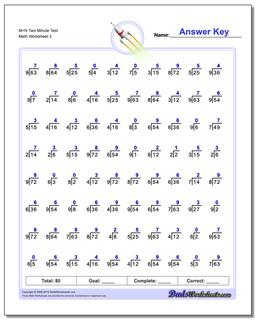One Dad. Four daughters. 9,251 worksheets... and counting!M+N Two Minute Test (Third Worksheet)

PropertyValue
DescriptionM+N Two Minute Test: 80 problem division worksheets similar to RocketMath tests designed for two minute drills. Each of these division worksheets combines two levels from one minute tests, introducing four to five new division problems on each worksheet. (Third Worksheet)
Resource TypeWorksheet